# Non Verbal Reasoning - Analytical Reasoning

Exercise : Analytical Reasoning - Section 1
26.

Find the number of triangles in the given figure.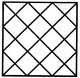28
32
36
40
Explanation:

The figure may be labelled as shown.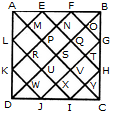The simplest triangles are AML, LRK, KWD, DWJ, JXI, IYC, CYH, HTG, GOB, BOF, FNE and EMA i.e. 12 in number.

The triangles composed of two components each are AEL, KDJ, HIC and FBG i.e. 4 in number.

The triangles composed of three components each are APF, EQB, BQH, GVC, CVJ, IUD, DUL and KPA i.e. 8 in number.

The triangles composed of six components each are ASB, BSC, CSD, DSA, AKF, EBH, CGJ and IDL i.e. 8 in number.

The triangles composed of twelve components each are ADB, ABC, BCD and CDA i.e. 4 in number.

Total number of triangles in the figure = 12 + 4 + 8 + 8 + 4 = 36.

27.

What is the number of triangles that can be formed whose vertices are the vertices of an octagon but have only one side common with that of octagon?

64
32
24
16
Explanation: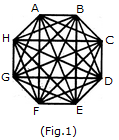When the triangles are drawn in an octagon with vertices same as those of the octagon and having one side common to that of the octagon, the figure will appear as shown in (Fig. 1).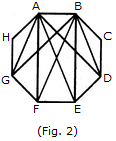Now, we shall first consider the triangles having only one side AB common with octagon ABCDEFGH and having vertices common with the octagon (See Fig. 2).Such triangles are ABD, ABE, ABF and ABG i.e. 4 in number.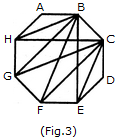Similarly, the triangles having only one side BC common with the octagon and also having vertices common with the octagon are BCE, BCF, BCG and BCH (as shown in Fig. 3). i.e. There are 4 such triangles.

This way, we have 4 triangles for each side of the octagon. Thus, there are 8 x 4 = 32 such triangles.

28.

Find the number of triangles in the given figure.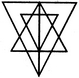27
25
23
21
Explanation:

The figure may be labelled as shown.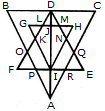The simplest triangles are GLK, DLJ, DJM, HMN, QRE, IRA, IPA and FPO i.e. 8 in number.

The triangles having two components each are BDO, CDQ, DLM, PRA, KFI, NEI, HJI, GJI, DKI and DNI i.e. 10 in number.

The triangles having four components each are DIE, DFI, DOA, DQA andGHI i.e. 5 in number.

The triangles having six components each are DCA and DBA i.e. 2 in number.

DEF is the only triangle having eight components.

ABC is the only triangle having twelve components.

Thus, there are 8+10+ 5 + 2+1 + 1 = 27 triangles in the figure.

29.

Find the number of triangles in the given figure.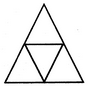4
5
6
7
Explanation:

The figure may be labelled as shown.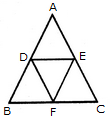The simplest triangles are ADE, BDF, DEF and EFC i.e. 4 in number.

There is only one triangle ABC composed of four components.

Thus, there are 4+1 = 5 triangles in the given figure.

30.

Find the number of triangles in the given figure.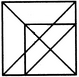16
18
19
21
Explanation:

The figure may be labelled as shown.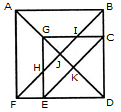The simplest triangles are EFH, BIC, GHJ, GIJ, EKD and CKD i.e. 6 in number.

The triangles composed of two components each are ABJ, AFJ, GCK, GEK, CED arid GHI i.e. 6 in number.

The triangles composed of three components each are GCD, GED, DJB and DJF i.e. 4 in number.

The triangles composed of four components each are ABF and GCE i.e. 2 in number.

The triangles composed of five components each are ABD and AFD i.e. 2 in number.

There is only one triangle i.e. FBD composed of six components.

Total number of triangles in the figure = 6 + 6 + 4 + 2 + 2 + 1 = 21.